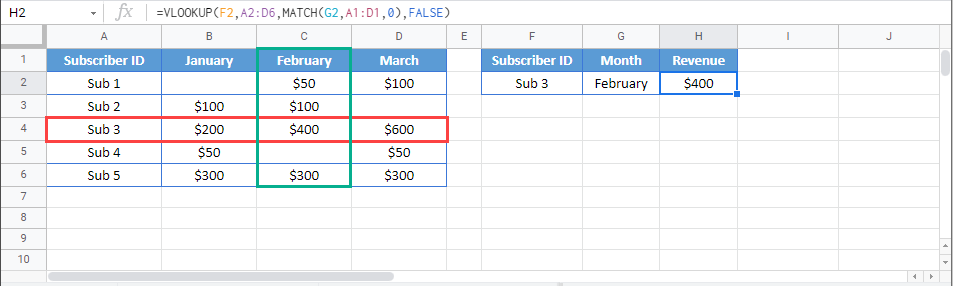# VLOOKUP – Dynamic Column Reference – Excel & Google Sheets

This tutorial will demonstrate how to perform a VLOOKUP with a dynamic column references using the VLOOKUP and MATCH Functions in Excel and Google Sheets. If you have access to the XLOOKUP Function, we recommend using a Dynamic XLOOKUP instead.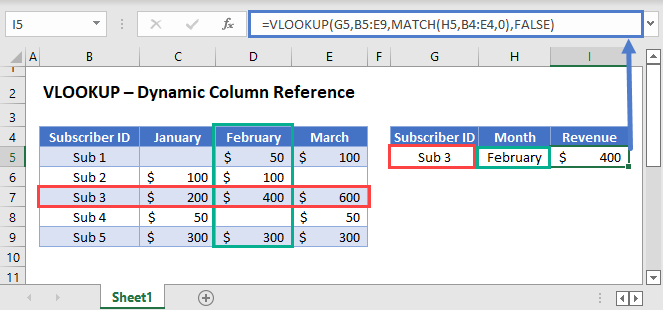## VLOOKUP Row and Column Coordinates

In general, all lookup processes require at least two things: the row and column coordinates of the value that will be looked up. With VLOOKUP, the row coordinate is determined by finding the lookup_value in the table_array. The col_index (3rd argument) provides column coordinate.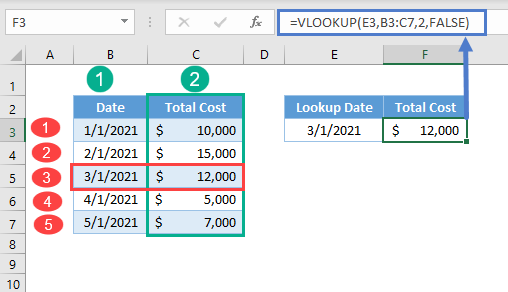## VLOOKUP with Match Function

Typically, we use the VLOOKUP Function with a constant column index number (as shown above). Instead, we can use a MATCH Function to calculate the column number.

``=VLOOKUP(G3,B3:E7,MATCH(H3,B2:E2,0),FALSE)``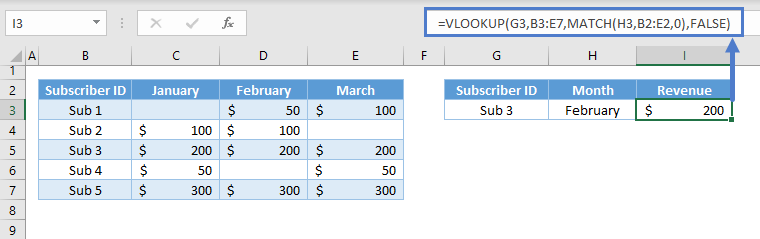Let’s walkthrough the formula:

### Match Function

First, use the MATCH Function to look up the correct column number.

``=MATCH(G3,B2:E2,0)``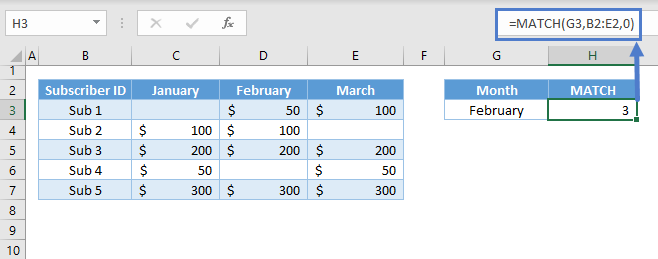Here we look up “February” in row 2 and find that “February” is found in the 3rd column of the table.

### VLOOKUP Function

Next, we’ll use the result of the MATCH Function as the input for the column index of the VLOOKUP Function: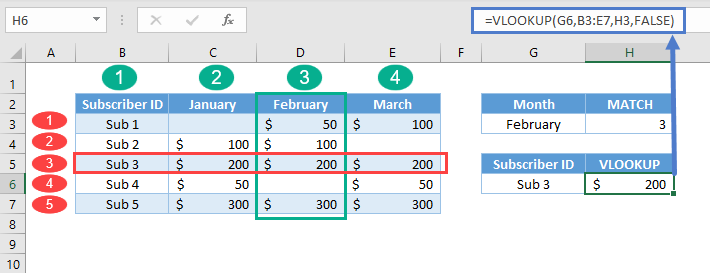Putting this all together, we get our original formula:

``=VLOOKUP(G3,B3:E7,MATCH(H3,B2:E2,0),FALSE)``

In summary, the formula performs two lookups, which are in vertical and horizontal directions: one from VLOOKUP (vertical lookup) and another from the MATCH Function (horizontal lookup); hence, it’s called a two-way lookup. An alternative to this formula is the INDEX-MATCH-MATCH combination.